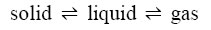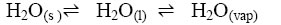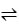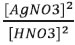# Equilibrium Constant KP Formula Chemical Ionic Consumer in PDF

Equilibrium Constant KP Formula Chemical Ionic Consumer In PDF

Chapter 7 Equilibrium

## Equilibrium Constant KP Formula Chemical Ionic Consumer in PDF

➣ Equilibrium state- When rate of formation of a product in a process is in competition with rate of formation of reactants, the state is then named as “Equilibrium state”.

➣  Equilibrium in physical processes:Law of chemical equilibrium: At a given temperature, the product
of concentrations of the reaction products raised to the respective stoichiometric coefficient in the balanced chemicalequation divided by the product ofconcentrations of the reactants raised totheir individual stoichiometric coefficientshas a constant value. This is known asthe Equilibrium Law or Law of Chemical Equilibrium.

 aA +bBcC + dD Kc =[C]c [D]d[A]a [B]b Chemical equation Equilibrium constant aA + b Bc C + D K) cC + d Da A + b B K' c=(1/Kc na A + nb B ncC + ndD K"" c= (Kcn)

Concentrations or partial pressure of pure solids or liquids do not appear in the expression of the equilibrium constant. In the reaction, KcAg2O(s) + 2HNO3M(aq)2AgNO3 (aq)+H2O(l)➣  If Qc >Kc, the reaction will proceed in the direction of reactants (reverse
reaction).If Qc <Kc, the reaction will proceed in the direction of the products (forward reaction)

➣  Kp is equilibrium constant in terms of partial pressure of gaseous reactants and products.

➣  Kc  is  equilibrium  constant  in  terms  of  molar  concentration  of  gaseous reactants and products

➣ Kp =Kc (RT)∆n here R is gas constant, T is temperature at which theprocess is carried out &∆n is no. of moles of gaseous product minus no. of moles of gaseous reactants.

➣  If Kc> 103;     Kc is very high i.e. the reaction proceeds nearly to completion.

➣  If Kc<103;     Kc is very small i.e. the reaction proceeds rarely.

If Kcis ranging in the range of 103 to 10-3;  i.e. reactants and products are just in equilibrium.

ΔGo = – RT lnK     or ΔGo = – 2.303RT log K

➣ Factors affecting equilibrium constant :- temperature, pressure, catalyst and molar concentration of reactants and products.

L e Chate lie r ’s   pr i nc i ple  :-  It  states that  a change in  any  of  the  factors that determine the equilibrium conditions of a system will cause the system to change in such a manner so as to reduce or toc ounteract the effect of the change.

Arrhenius acids are the substances that ionize in water to form H+ .

Arrhenius bases are the substances that ionize in water to form OH-.

Lewis acids are lone pair (of e-) accepters while Lewis bases are lone pair donators.

Proton donor are acids while proton accepters are bases(Bronsted-Lowry concept).

The acid-base pair thatdiffers only by one proton is called a conjugateacid- base pair. IfBrönsted acid is a strong acid then itsconjugate base is a weak base and viceversa.

Ionic product of water.Kw = [H+][OH]
pH = -log [H+] ; here[H+] is molar concentration of hydrogen ion.
pH + pOH =14
pKa + pKb =14
Ka x Kb = Kw = ionic product of water=1 x 10-14

Buffer solution  :The solutions which resist change in pH on dilution or with the addition of small amounts of acid or alkali are called Buffer Solutions.

common ion effect: It can be defined as a shift in equilibrium on adding a substance  that  provides  more  of  an  ionic  species  already  present  in  the dissociation equilibrium.

➣ Hydrolysis of Salts: process of interaction between water and cations an ions or both of salts is call edhydrolysis.

The cations (e.g., Na+, K+,Ca2+, Ba2+, etc.) of strong bases and anions(e.g., Cl, Br, NO3–, ClO4– etc.) of strong acids simply get hydrated but do not hydrolyse, and therefore the solutions of salts formed from strong acids and bases are neutral i.e., theirpH is 7.

Salts of weak acid and strong base e.g., CH3COON a are basic in nature.

Salts of strong acid and weak base e.g.,NH4Cl, are acidic

Salts of weak acid and weak base, e.g.,CH3COONH4. The pH is determined by the formula    pH = 7 + ½ (pKa – pKb)

Solubility  product-  product  of  the  molar  concentrations  of  the  ions  in  a saturated solution, each concentration term raised to the power equal to the no. of ions produced.

Chapter 7 part -1Chapter 7 Part 2

### NCERT Books Free Pdf Download for Class 5, 6, 7, 8, 9, 10 , 11, 12 Hindi and English Medium

 Mathematics Biology Psychology Chemistry English Economics Sociology Hindi Business Studies Geography Science Political Science Statistics Physics Accountancy